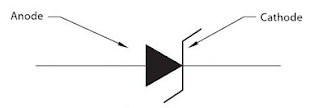### Zener Diodes

Zener diodes are special diodes that have a low reverse voltage break down rating. They are named for C.A. Zener, who analyzed the voltage breakdown of electrical insulators. Zener diodes generally have a reverse breakdown voltage of about 3-200 volts. They are intended to be operated with current flowing through them in the reverse direction. The symbole for a zener diode is shown in the Fig. 1.Fig. 1 Zener diode symbole
When the reverse breakdown voltage of a diode is exceeded, the current suddenly begins to flow with almost no restriction. This is generally referred to as avalanche condition. Zener diodes are sometimes referred to as avalanche diodes. This avalanche current is referred to as the zener region, Figure 2. Notes in Figure 2 that the reverse voltage drop is relatively constant over a wide range of current. Diodes are not harmed when they operate in the zener region as long as their electrical values of current or watts are not exceeded.Fig. 2 The current avalanches in the reverse direction
Because the voltage drop across the zener diode is constant, the diode is generally used as a voltage regulator. Any device or devices connected in parallel with the zener will have the same voltage applied.
An example circuit is shown in Figure 3. The zener diode has a reverse voltage rating of 12 volts and a power rating of 5 watts. Resistor R1 is used to limit the current in the circuit. Resistors R2 and R3 represent the load for the circuit. Note the supply voltage must be greater than the zener voltage for the circuit to operate.Fig. 3 Zener diode used as voltage regulator
Resistor R1 and the zener diode form a series circuit to ground. Because the zener has a constant voltage drop of 12 volts,resistor R1 will have a voltage drop of 8 volts (20 volts - 12 volts = 8 volts). Resistor R1, therefor will permit a total current flow of 0.08 A (80mA) in this circuit (8 volts /100 Ω = 0.08 A).
The load circuit, R2 and R3, is connected in parallel with the zener diode. Because the load is in parallel with the zener, the voltage dropped across the zener will be applied to the load. If the zener maintains a constant voltage drop of 12 volts, the load will have a constant applied voltage of 12 volts. The maximum current that can flow through the load is 0.06 A (60 mA), (12 volts/200 Ω = 0.06 A). Note that the value of R1 was chosen to ensure that there would be sufficient current to operate the load. In order for a load current of 60 mA to flow, the value of R3 is adjusted to 0 Ω. At this point ammeter A2 would indicate a flow of 60 mA and ammeter A1 would indicate 20 mA. The values of A1 and A2 will always add to equal the maximum current that can flow through the circuit, (60 mA + 20mA = 80 mA). Voltmeter will indicate a value of 12 volts.
Now assume that resistor R3 is adjusted to a value of 200 ohms. The load now has a resistance of 400 Ω (200 Ω + 200 Ω = 400 Ω). Ammeter A2 will now indicate a current flow of 30 mA (12 volts/400 Ω = 0.030). Ammeter A1, however, now indicates a current flow of 50 mA (50 mA + 30 mA = 80 mA). Voltmeter V1 indicates a value of 12 volts.
Zener diodes can be tested in the same manner as rectifier diodes provided the ohmmeter voltage is less than the reverse voltage value of the zener. For example,an ohmmeter that provided an output voltage of 6 volts could not be used to test a zener diode with a reverse voltage rating of 5 volts. The zener diode would appear to be shorted even if it were not.
Zener Diode Application
A good example of how a zener diode can be used as a voltage regulator can be found in the charging circuit of many motorcycles. Figure 4 In this circuit, the alternator is used to produce the direct current needed to operate the electrical system of the motorcycle and charge the battery. When a 12-volt battery is fully charged, it will exhibit a voltage of 12 volts across its terminals. If the voltage supplied to the battery becomes greater than 14 volts, there is danger of overcharging the battery.Fig. 4 Typical voltage regulator circuit for a motorcycle

In this circuit, the alternator is connected in parallel with the battery. A power zener diode is connected in parallel with both the alternator and battery. Because electrical components connected in parallel must have the same voltage, the zener diode will not permit the voltage supplied to the battery to become greater than 14 volts. Notice that there is no current limiting resistor connected in series with the zener diode. The current in this circuit is limited by the internal impedance of the alternator.